Question

A square conducting loop with side length

a = 1.25 cm

is placed at the center of a solenoid 40.0 cm long with a current of 4.60 A flowing through its 470 turns, and it is aligned so that the plane of the loop is perpendicular to the long axis of the solenoid. The radius of the solenoid is 5.00 cm.

(a) What is the magnetic flux through the loop?
Wb

(b) What is the magnitude of the average emf induced in the loop if the current in the solenoid is increased from 4.60 A to 20.0 A in 1.75 s?
V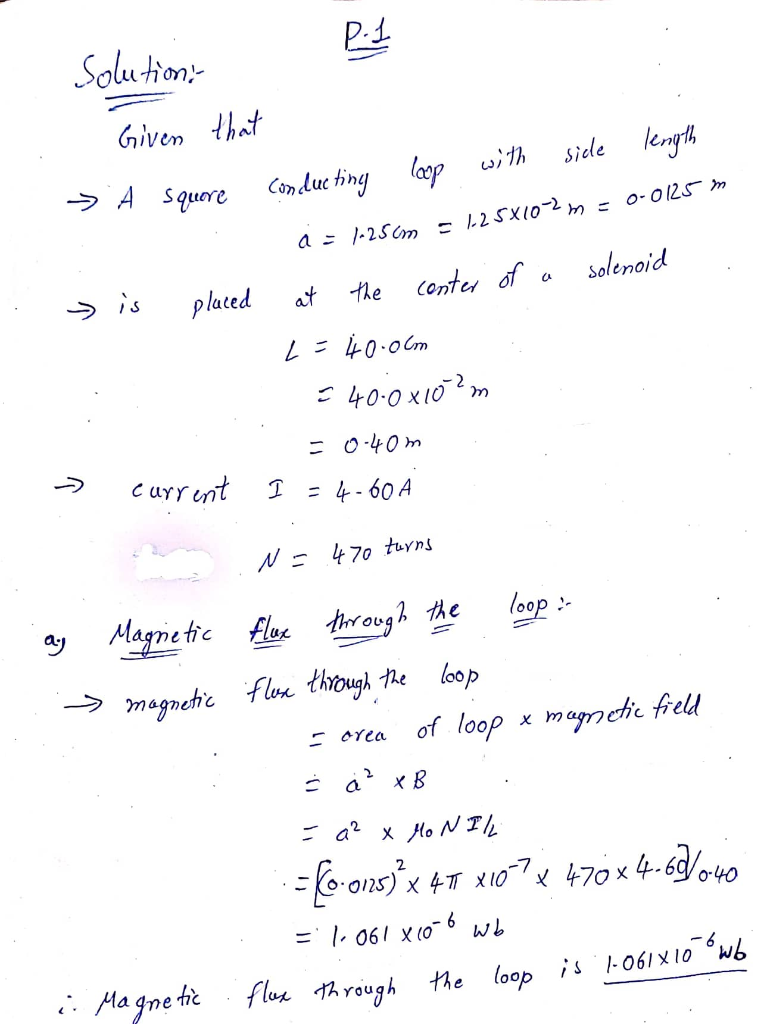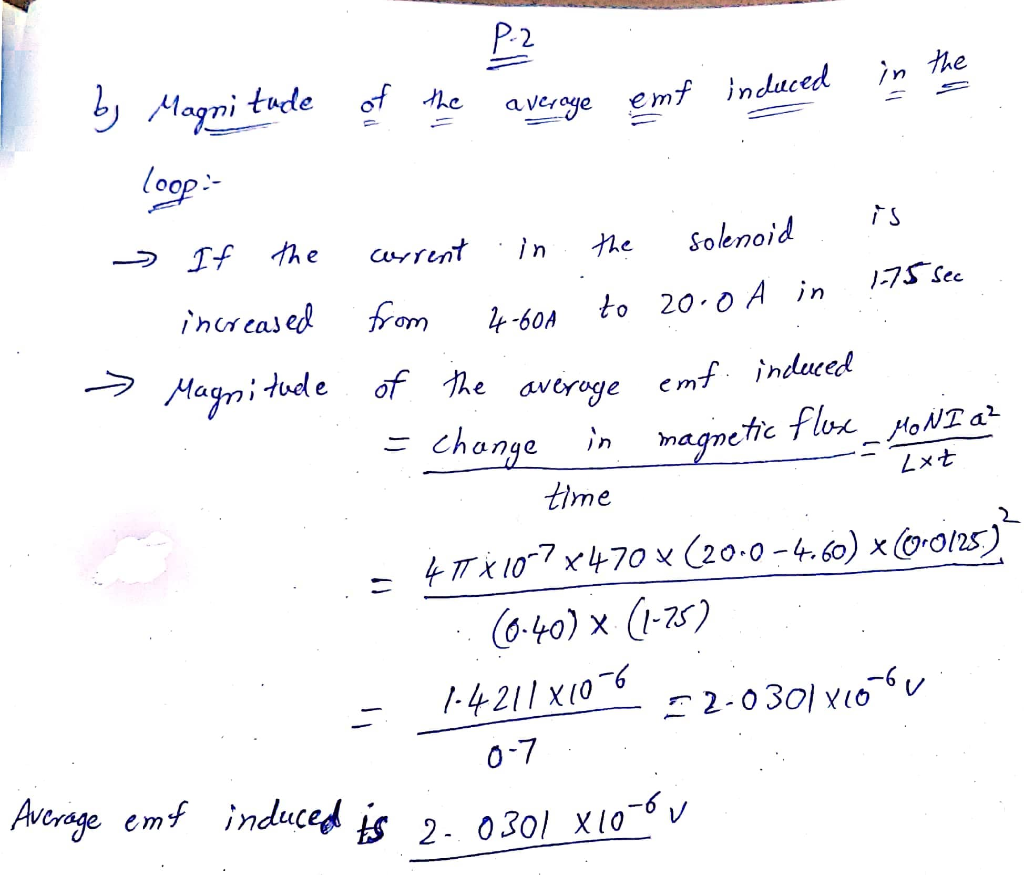#### Earn Coins

Coins can be redeemed for fabulous gifts.

Similar Homework Help Questions
• ### A single-turn square loop of side L is centered on the axis of a long solenoid....

A single-turn square loop of side L is centered on the axis of a long solenoid. In addition, the plane of the square loop is perpendicular to the axis of the solenoid. The solenoid has 1310 turns per meter and a diameter of 4.00 cm, and carries a current of 2.41 A. (a) Find the magnetic flux through the loop when L = 2 cm. Wb (b) Find the magnetic flux through the loop when L = 4.00 cm. Wb...

• ### A square, single-turn wire loop ℓ = 1.00 cm on a side is placed inside a...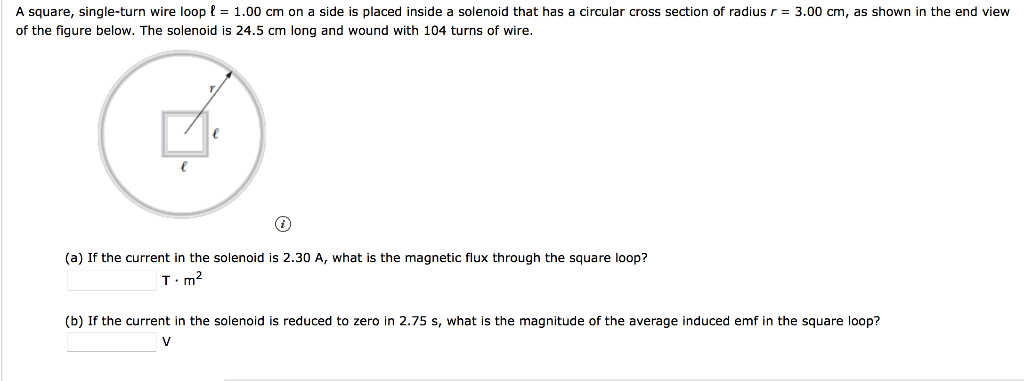A square, single-turn wire loop ℓ = 1.00 cm on a side is placed inside a solenoid that has a circular cross section of radius r = 3.00 cm, as shown in the end view of the figure below. The solenoid is 24.5 cm long and wound with 104 turns of wire. (a) If the current in the solenoid is 2.30 A, what is the magnetic flux through the square loop? T · m2 (b) If the current in the...

• ### A square, single-turn wire loop ℓ = 1.00 cm on a side is placed inside a...

A square, single-turn wire loop ℓ = 1.00 cm on a side is placed inside a solenoid that has a circular cross section of radius r = 3.00 cm, as shown in the end view of the figure below. The solenoid is 24.0 cm long and wound with 122 turns of wire. (a) If the current in the solenoid is 2.35 A, what is the magnetic flux through the square loop? T · m2 (b) If the current in the...

• ### 2. 5 points KatzPSEI1 31 P040. My Notes Ask Your Teach A square conducting loop with...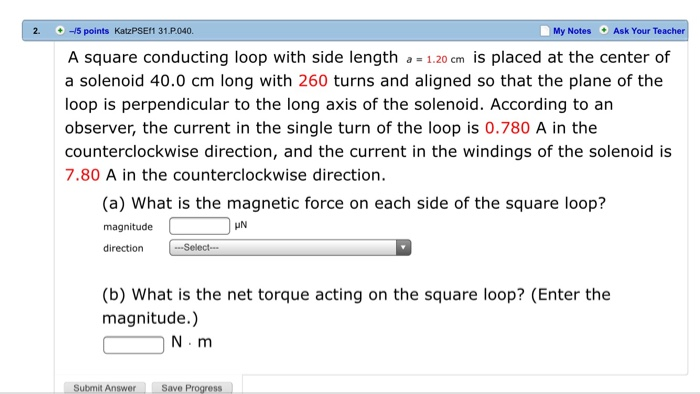2. 5 points KatzPSEI1 31 P040. My Notes Ask Your Teach A square conducting loop with side length 1.20 cm is placed at the center of a solenoid 40.0 cm long with 260 turns and aligned so that the plane of the loop is perpendicular to the long axis of the solenoid. According to an observer, the current in the single turn of the loop is 0.780 A in the counterclockwise direction, and the current in the windings of the...

• ### A square loop of wire with side 3 cm is placed in a uniform magnetic field...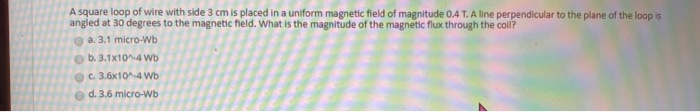A square loop of wire with side 3 cm is placed in a uniform magnetic field of magnitude 0.4 T. A line perpendicular to the plane of the loop is angled at 30 degrees to the magnetic field. What is the magnitude of the magnetic flux through the coll A square loop of wire with side 3 cm is placed in a uniform magnetic field of magnitude 0.4 T. A line perpendicular to the plane of the loop is angled...

• ### A solenoid with 1200 turns per meter has a diameter of 4.00 cm. A current I...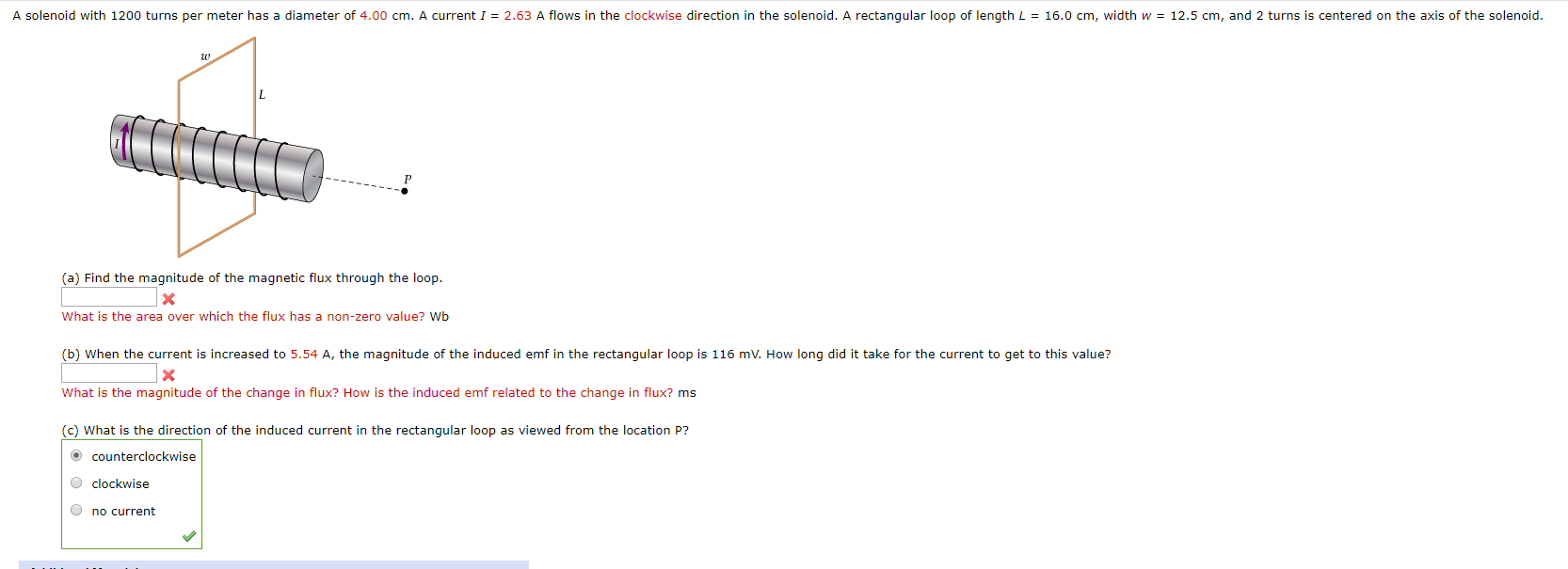A solenoid with 1200 turns per meter has a diameter of 4.00 cm. A current I = 2.63 A flows in the clockwise direction in the solenoid. A rectangular loop of length L = 16.0 cm, width w = 12.5 cm, and 2 turns is centered on the axis of the solenoid. (a) Find the magnitude of the magnetic flux through the loop. What is the area over which the flux has a non-zero value? Wb (b) When the current...

• ### A solenoid of radius r = 1.25 cm and length ℓ = 32.0 cm has 295...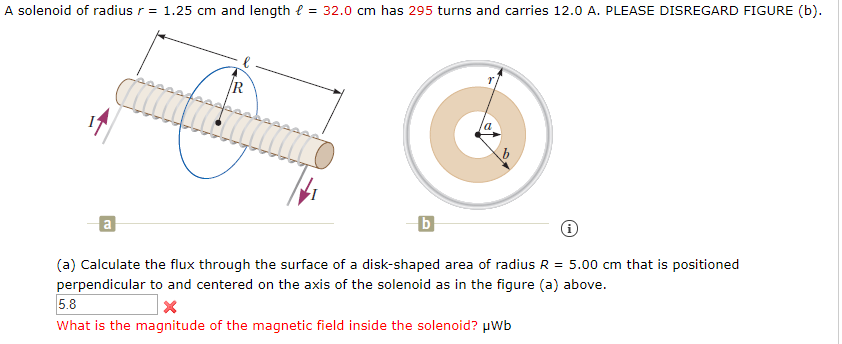A solenoid of radius r = 1.25 cm and length ℓ = 32.0 cm has 295 turns and carries 12.0 A. PLEASE DISREGARD FIGURE (b) A solenoid of radius r = 1.25 cm and length t = 32.0 cm has 295 turns and carries 12.0 A. PLEASE DISREGARD FIGURE (b). AAAA (a) Calculate the flux through the surface of a disk-shaped area of radius R = 5.00 cm that is positioned perpendicular to and centered on the axis of the...

• ### A square loop with a single turn, with side L= 0.45cm is placed inside a solenoid...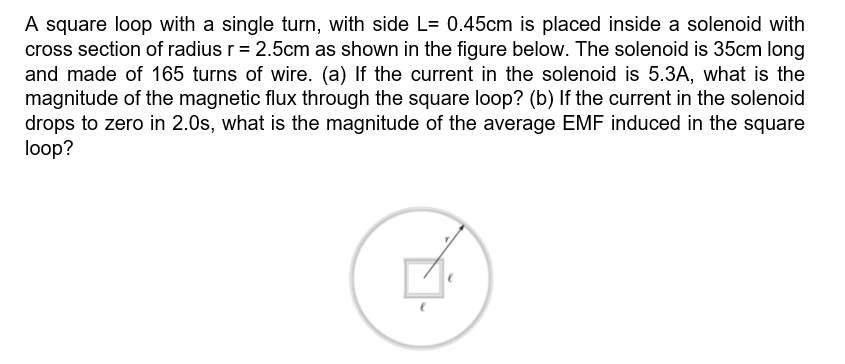A square loop with a single turn, with side L= 0.45cm is placed inside a solenoid with cross section of radius r = 2.5cm as shown in the figure below. The solenoid is 35cm long and made of 165 turns of wire. (a) If the current in the solenoid is 5.3A, what is the magnitude of the magnetic flux through the square loop? (b) If the current in the solenoid drops to zero in 2.0s, what is the magnitude of...

• ### A single-turn square loop of side L is centered on the axis of a long solenoid....

A single-turn square loop of side L is centered on the axis of a long solenoid. In addition, the plane of the square loop is perpendicular to the axis of the solenoid. The solenoid has 1310 turns per meter and a diameter of 5.55 cm , and carries a current of 2.55 A . Find the magnetic flux through the loop when L= 2.95 cm .

• ### A square, single-turn wire loop ! - 1.00 cm on a side is placed inside a...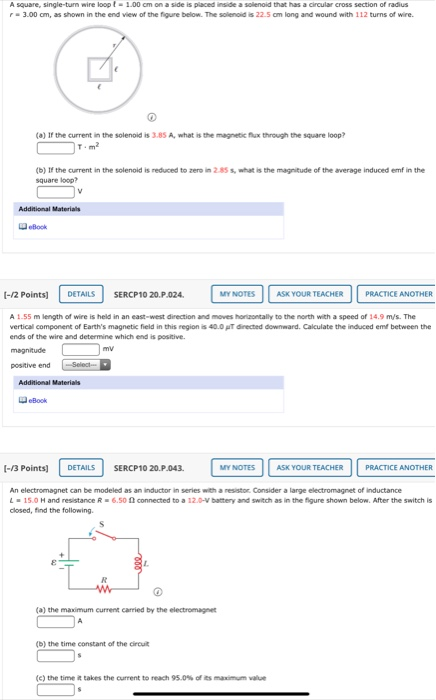A square, single-turn wire loop ! - 1.00 cm on a side is placed inside a solenoid that has a circular cross section of radius - 3.00 cm, as shown in the end view of the figure below. The solenoid is 22.5 cm long and wound with 112 turns of wire. (a) If the current in the solenoid is 3.85 A, what is the magnetic flux through the square loop? (b) If the current in the solenoid is reduced to...